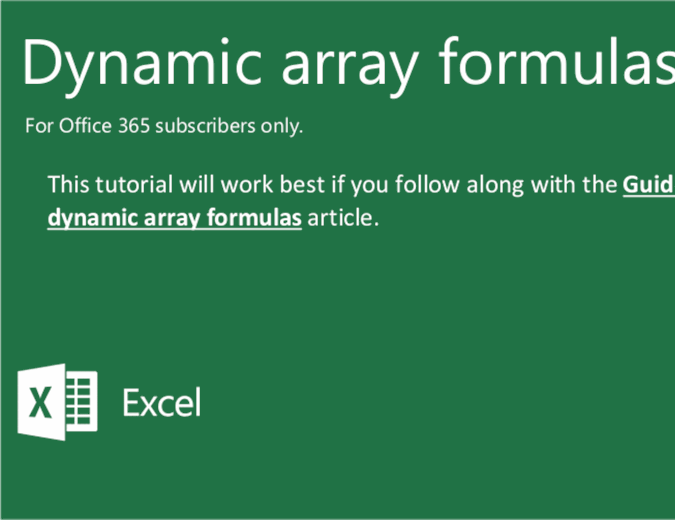Go back to school with Office templates, designed for school activities and classroom safety. DOWNLOAD THE TEMPLATES NOW.

# Dynamic array formulas tutorial

Become an Excel power user and learn about array formulas with the help of this formulas tutorial template. Use this dynamic array formulas tutorial template to complete complex tasks in Excel. This array formulas tutorial template will teach you many efficient tips in Excel, such as calculating the cost of a loan and much more.

Excel